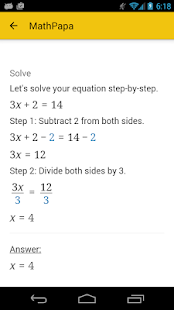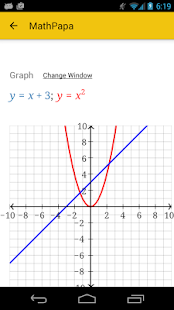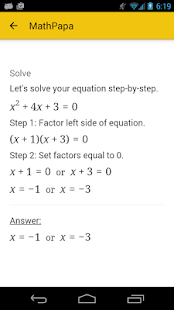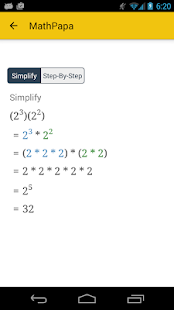MathPapa – Algebra Calculator

1.0.7Download APK
Developer
MathPapa, Inc.
Version
1.0.7
Updated
December 31, 2018
Requirements
4.4 and up

Description

SOLVE your algebra problems step-by-step with MathPapa!MathPapa can solve your equations (and show the work!) and help you when you’re stuck on your math homework.FEATURES:• Solves linear equations and quadratic equations.• Solves linear and quadratic inequalities.• Graphs equations.• Factors quadratic expressions.• Order of operations step-by-step.• Evaluates expressions.• Solves systems of two equations.WORKS OFFLINE!STEP-BY-STEP SOLUTIONS:MathPapa’s goal is to help you learn algebra step-by-step.Get help on your algebra problems with the Math Papa Algebra Calculator!HOW TO USE THE CALCULATOR:Just type your problem into the text box.For example, enter 3x+5=17 into the text box to get a step-by-step explanation of how to solve 3x+5=17.MATH SYMBOLS:Here are some symbols that the Math Papa calculator understands:+ (Addition) – (Subtraction) * (Multiplication) / (Division) ^ (Exponent: “raised to the power”) √ (Square Root)|x| (Absolute Value of x)Privacy Policy: https://www.mathpapa.com/privacy/Terms of Use: https://www.mathpapa.com/terms/
Minor update

Minor update

Images